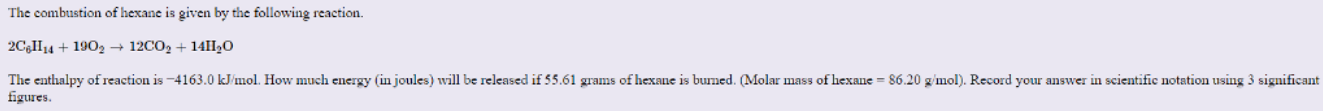# Problem: The combustion of hexane is given by the following reaction. 2C6H14 + 19O2 --&gt; 12CO2 + 14H2O The enthalpy of reaction is -4163.0 kJ/mol. How much energy (in joules) will be released if 55.61 grams of hexane is burned. (Molar mass of hexane = 86.20 g/mol). Record your answer in scientific notation using 3 significant figures.

###### FREE Expert Solution
100% (340 ratings)###### Problem Details

The combustion of hexane is given by the following reaction. 2C6H14 + 19O2 --> 12CO2 + 14H2O The enthalpy of reaction is -4163.0 kJ/mol. How much energy (in joules) will be released if 55.61 grams of hexane is burned. (Molar mass of hexane = 86.20 g/mol). Record your answer in scientific notation using 3 significant figures.